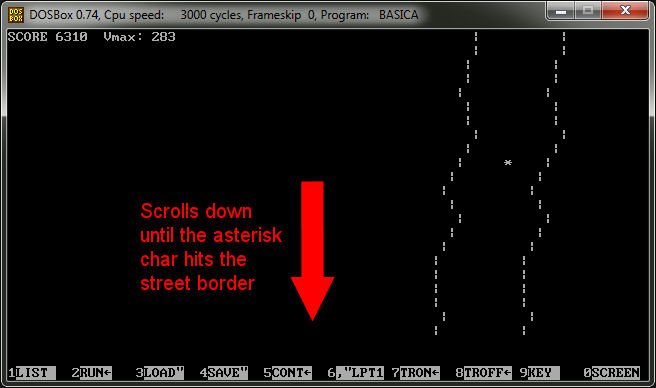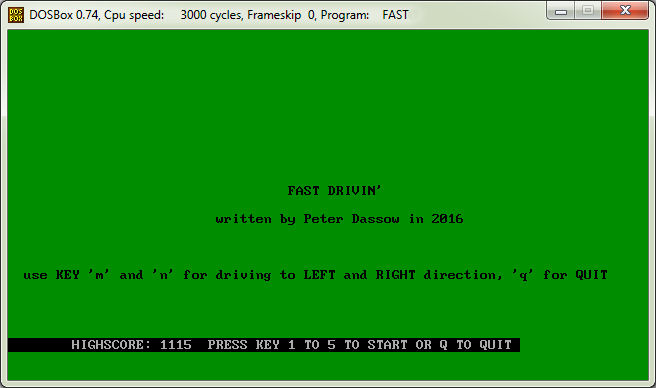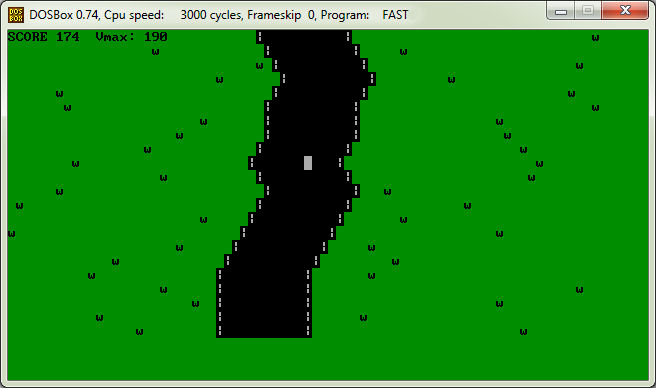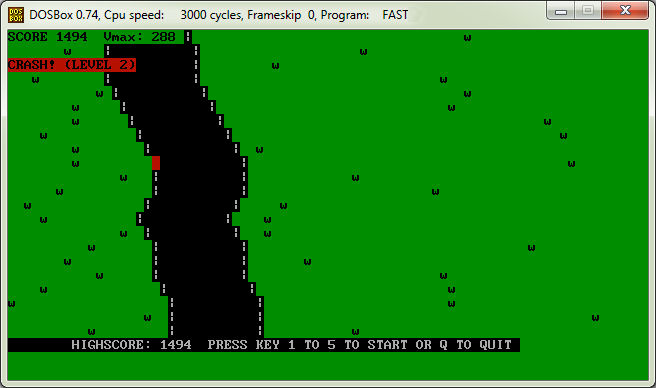Peter's z80.eu site blog
Search

Please note also - THIS BLOG ENDS HERE WITH THE LAST ENTRY FROM NOVEMBER 9th 2021.

I have prepared a new blog with wordpress at https://vintagecomputing.info !!!

Thank you.
Programming a BASIC game - still fun, even with a vintage PC/XT
Tuesday, March 15, 2016, 11:00 PM
Yes, it can be still fun, although you should not try to program too large programs in BASIC.

I remembered these racing game tries of the first microcomputer days, without any high res graphics, just characters. So I tried to program a small game in less than 1KB source code.
That's the result:

10 DEFINT A-Z:RANDOMIZE TIMER:D!=TIMER:S=RND*40!+20:C=S+4:O=C:CLS
20 LOCATE 11,1:FOR I=1 TO 12:PRINT SPC(S);"|";SPC(10);"|":NEXT I:Z=0:V!=.3
30 R=RND*7:IF R<3 THEN M=-1 ELSE IF R=3 THEN M=0 ELSE M=1
40 LOCATE 2,1:PRINT "SCORE";Z;" Vmax:";310-CINT(V!*1000!):S=S+M
50 LOCATE 23,1:IF S>58 THEN S=58 ELSE IF S<1 THEN S=1
60 PRINT SPC(S%);"|";SPC(10);"|":PRINT:Z=Z+10:T=SCREEN(10,C):LOCATE 10,C
70 PRINT "*";:LOCATE 9,O:PRINT " ";:IF T<>32 THEN LOCATE 3,1:PRINT "CRASH!"
80 IF T<>32 THEN SOUND 37,5:LOCATE 23,1:END ELSE O=C:IF TIMER<D!+1 THEN 110
90 IF V!>.1 THEN V!=V!-.01 ELSE IF V!>.05 THEN V!=V!-.005 ELSE V!=V!-.001
100 D!=TIMER:SOUND Z/10+100,2:IF V!<0 THEN V!=0
110 K\$=INKEY\$:T!=TIMER+V!:WHILE TIMER<T!:WEND
120 IF K\$="" THEN 30 ELSE IF K\$="n" THEN C=C-1 ELSE IF K\$="m" THEN C=C+1
130 IF K\$="q" THEN LOCATE 23,1:END ELSE 30:REM WRITTEN BY P. DASSOW

And that's how it looks:If you would say "that's not coded perfectly", that's true, but some strange things like two IF branches with the same condition - see line 70 and 80 - are done just because to get less lines and having still a line length < 80 chars. It is programmed CPU speed "independent" (means delays are timer based).

This is a version with more lines, but somewhat speed optimized:

10 DEFINT A-Z:RANDOMIZE TIMER:D!=TIMER:S=RND*40!+20:C=S+4:W\$="|"+SPACE\$(10)+"|"
20 CLS:O=C:LOCATE 11,1:FOR I=1 TO 12:PRINT SPC(S);W\$:NEXT I:Z=0:V!=.3
30 R=RND*7:IF R<3 THEN M=-1 ELSE IF R=3 THEN M=0 ELSE M=1
40 LOCATE 2,1:PRINT "SCORE";Z;" Vmax:";310-CINT(V!*1000!):S=S+M
50 LOCATE 23,1:IF S>58 THEN S=58 ELSE IF S<1 THEN S=1
60 PRINT SPC(S%);W\$:PRINT:Z=Z+10:T=SCREEN(10,C):LOCATE 10,C
70 PRINT "*";:LOCATE 9,O:PRINT " ";:IF T=32 THEN O=C:IF TIMER<D!+1 THEN 110 ELSE 90
80 LOCATE 3,1:PRINT "CRASH!":SOUND 37,5:LOCATE 23,1:END
90 IF V!>.1 THEN V!=V!-.01 ELSE IF V!>.05 THEN V!=V!-.005 ELSE V!=V!-.001
100 D!=TIMER:SOUND Z/10+100,2:IF V!<0 THEN V!=0
110 K\$=INKEY\$:T!=TIMER+V!:WHILE TIMER<T!:WEND
120 IF K\$="" THEN 30
130 IF K\$="n" THEN C=C-1:GOTO 30
140 IF K\$="m" THEN C=C+1:GOTO 30
150 IF K\$="q" THEN LOCATE 23,1:END
160 GOTO 30:REM WRITTEN BY P. DASSOW

Now imagine to spend additional 4 hours of testing and programming, and see the results:I added a title screen with explanations, a bit more color, some random grass, a more sophisticated curve algorithm to have a more sinuos course, and also high score saving.

There is still a problem with the TIMER variable, if speed reaches the value 307, subtracting TIMER with very small values will result in inaccurate behaviour of the car on the street (it warps forward until it crashes).
This is surprisingly NOT the case if you execute the program on a slow PC/XT or an AMSTRAD PC 1640 for example. On such a slow computer, you will reach the highest possible speed of 310 without problems.... strange, isn't it ?

The versions:
The original first BASIC source code
The compiled first BASIC version, DOS compatible
The original first BASIC source code, optimized
The compiled and "improved" BASIC version, DOS compatible
The compiled and "improved" BASIC version, OS/2 compatible

Compiled versions are for 286 based PCs and older. Source can be run with an BASICA/GWBASIC interpreter from 386 up to a modern PC, if DOS (or DOSBOX) is used.

See related link for the WINDOWS 7 compatible QB64 compiled and "improved" version.
If you're curios about the source code of the "improved" version, please send me a mail, and you will get the source code (2.5 KB and still BASICA compatible) of it also.

<<First <Back | 15 | 16 | 17 | 18 | 19 | 20 | 21 | 22 | 23 | 24 | Next> Last>>